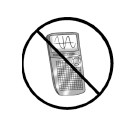### Home > A2C > Chapter 3 > Lesson 3.1.1 > Problem3-14

3-14.

Without using a calculator, perform each operation indicated in the expressions below.1. $\frac { 3 } { 4 } - \frac { 2 } { 5 }$

Refer to problem 2-89.

1. $\frac { 3 } { y } - \frac { 5 } { 4 }$

See part (a).

1. $( \frac { 3 m } { n } ) \cdot ( \frac { m } { 6 n } )$

$\frac{\textit{m}^{2}}{2\textit{n}^{2}}$

d.$( \frac { 5 x ^ { 2 } } { y } ) \cdot ( \frac { 10 } { x } )$

$\frac{50\textit{x}}{\textit{y}}$